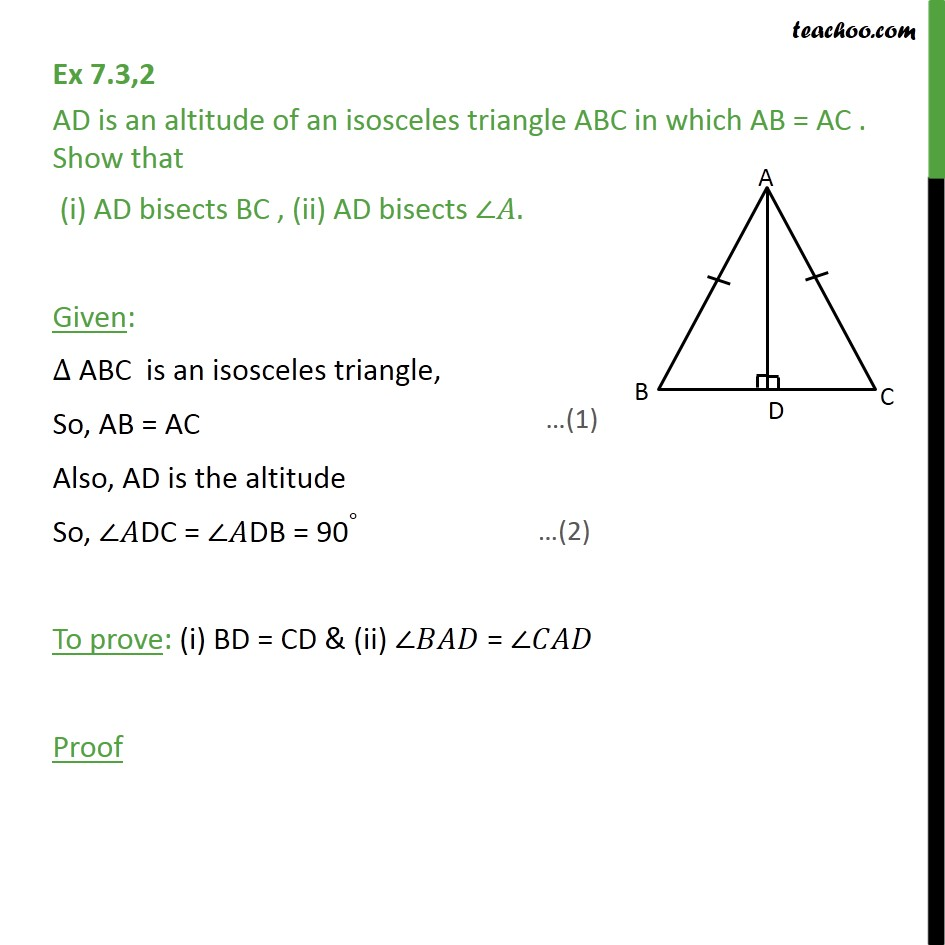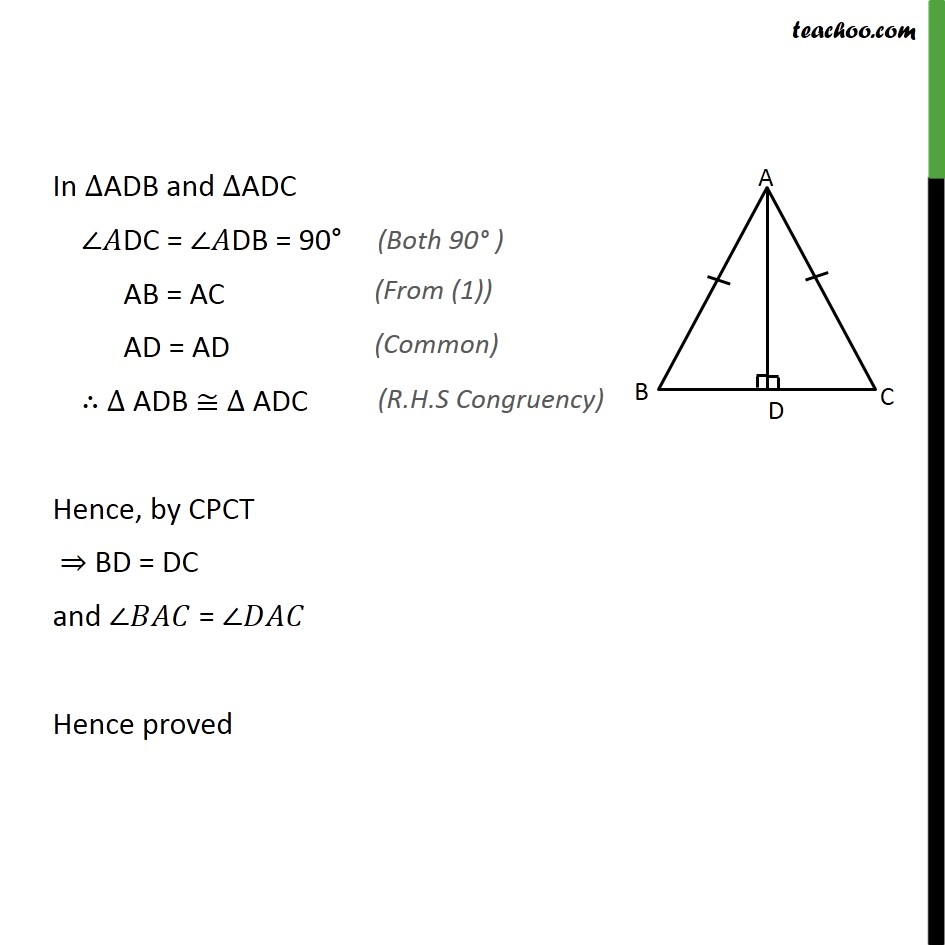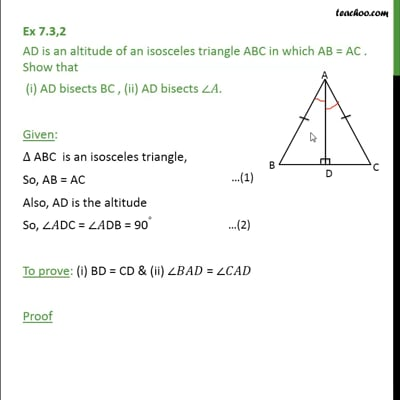Ex 7.3

Chapter 7 Class 9 Triangles
Serial order wiseThis video is only available for Teachoo black users

Maths Crash Course - Live lectures + all videos + Real time Doubt solving!

### Transcript

Ex 7.3,2 AD is an altitude of an isosceles triangle ABC in which AB = AC . Show that (i) AD bisects BC , (ii) AD bisects ∠𝐴. Given: ∆ ABC is an isosceles triangle, So, AB = AC Also, AD is the altitude So, ∠𝐴DC = ∠𝐴DB = 90∘ To prove: (i) BD = CD & (ii) ∠𝐵𝐴𝐷 = ∠𝐶𝐴𝐷 Proof In ∆ADB and ∆ADC ∠𝐴DC = ∠𝐴DB = 90° AB = AC AD = AD ∴ ∆ ADB ≅ ∆ ADC Hence, by CPCT ⇒ BD = DC and ∠𝐵𝐴𝐶 = ∠𝐷𝐴𝐶 Hence proved